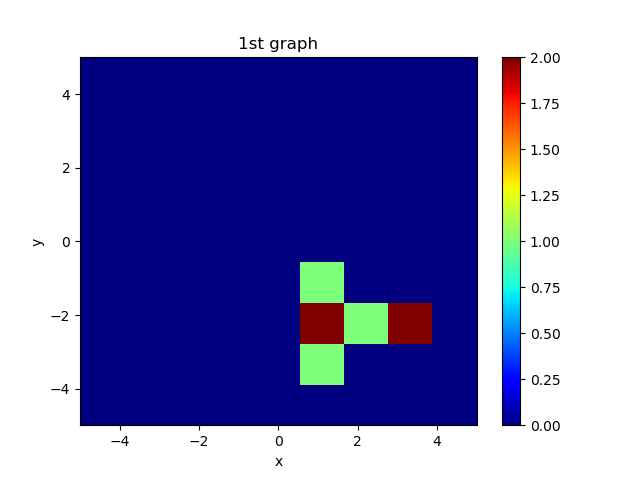# python --Export 2D histogram as an array by Matplotlib

The method of drawing a two-dimensional histogram with python is famous using Matplotlib or OpenCV.

In this article, when you create a 2D histogram with Matplotlib, make a note of how to write the histogram as a 2D array to a text file.

## [Python] Two-dimensional histogram in Matplotlib

@Supersaiakujin's article is recommended for how to create a 2D histogram image using Matplotlib.

[Python] How to create a 2D histogram with Matplotlib

The outline is to create a 2D histogram by passing two numpy arrays that are the source data to the "hist2d" function of the "Matplotlib" library.

It should be noted that it is necessary to create "an array with only x-coordinate" and "an array with only y-coordinate" for the two numpy arrays that are the original data, respectively.Such a 2D histogram can be written with the following sample program.

#### `hist2d`

``````
import numpy as np
import matplotlib.pyplot as plt
import matplotlib.cm as cm

#raw data
x = (1.1,1.1,3.2,3.1,1.1,2.1,1.1)
y = (-1.1,-2.1,-2.1,-2.1,-3.1,-2.1,-2.1)

#Preparation for graph drawing
fig = plt.figure()

#Histogram creation hist2d(Array,Array,Specify drawing range(Lower limit, upper limit, number of divisions),Color bar shade specification)
H = ax.hist2d(x,y, bins=[np.linspace(-5,5,10),np.linspace(-5,5,10)], cmap=cm.jet)
ax.set_title('1st graph')
ax.set_xlabel('x')
ax.set_ylabel('y')
fig.colorbar(H,ax=ax)
plt.show()
``````

## Export 2D histogram values as an array

The save function of numpy is convenient for writing the values of the 2D histogram as an array to a text file or csv.

#### `savetxt`

``````
#Histogram creation hist2d(Array,Array,Specify drawing range(Lower limit, upper limit, number of divisions),Color bar shade specification)
H = ax.hist2d(x,y, bins=[np.linspace(-5,5,10),np.linspace(-5,5,10)], cmap=cm.jet)
np.savetxt("2Dhist-array.txt", H, fmt = "%s")
``````

If you check the return value of hist2d in the official Matplotlib manual, you can see that the first return value is the histogram value. Therefore, H  is taken out.

fmt = "% s" is a character string format specification.

hist2d
H Histogram value(A two-dimensional array)
H x-axis split value (one-dimensional array)
H Y-axis division value (one-dimensional array)

Matplotlib.pyplot.hist2d

``````#Histogram creation hist2d(Array,Array,Specify drawing range(Lower limit, upper limit, number of divisions),Color bar shade specification)
H = ax.hist2d(x,y, bins=[np.linspace(-5,5,10),np.linspace(-5,5,10)], cmap=cm.jet)
print(H)
>>>[[0. 0. 0. 0. 0. 0. 0. 0. 0.]
[0. 0. 0. 0. 0. 0. 0. 0. 0.]
[0. 0. 0. 0. 0. 0. 0. 0. 0.]
[0. 0. 0. 0. 0. 0. 0. 0. 0.]
[0. 0. 0. 0. 0. 0. 0. 0. 0.]
[0. 1. 2. 1. 0. 0. 0. 0. 0.]
[0. 0. 1. 0. 0. 0. 0. 0. 0.]
[0. 0. 2. 0. 0. 0. 0. 0. 0.]
[0. 0. 0. 0. 0. 0. 0. 0. 0.]]
``````

As far as the output array is seen, a one-dimensional array is generated along the y-axis.

I have put together this code.

``````import numpy as np
import matplotlib.pyplot as plt
import matplotlib.cm as cm

#raw data
x = (1.1,1.1,3.2,3.1,1.1,2.1,1.1)
y = (-1.1,-2.1,-2.1,-2.1,-3.1,-2.1,-2.1)

#Preparation for graph drawing
fig = plt.figure()